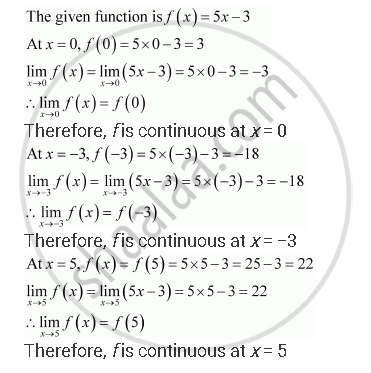Share

# Prove that the Function F (X) = 5x – 3 is Continuous at X = 0, at X = – 3 and at X = 5. - CBSE (Science) Class 12 - Mathematics

#### Question

Prove that the function f (x) = 5x – 3 is continuous at x = 0, at x = – 3 and at x = 5.

#### SolutionIs there an error in this question or solution?

#### APPEARS IN

NCERT Solution for Mathematics Textbook for Class 12 (2018 to Current)
Chapter 5: Continuity and Differentiability
Q: 1 | Page no. 159

#### Video TutorialsVIEW ALL 

Solution Prove that the Function F (X) = 5x – 3 is Continuous at X = 0, at X = – 3 and at X = 5. Concept: Concept of Continuity.
S